# College Chemistry : Properties of Gases

## Example Questions

### Example Question #1 : Gases

A gas does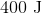of work while expanding and absorbs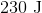of heat. What is the change in internal energy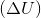?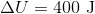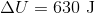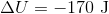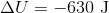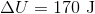Explanation:

The correct answer is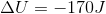.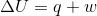where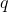represents heat and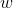represents work.

If a gas is expanding, it is doing work on the surroundings.  This means thatwill be a negative value. Because the gas is absorbing heat,entering the system and will be a positive value.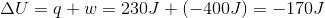### Example Question #2 : Gases

The graph shows molecular velocities for four different molecules at the same temperature. Which molecule has the highest molar mass?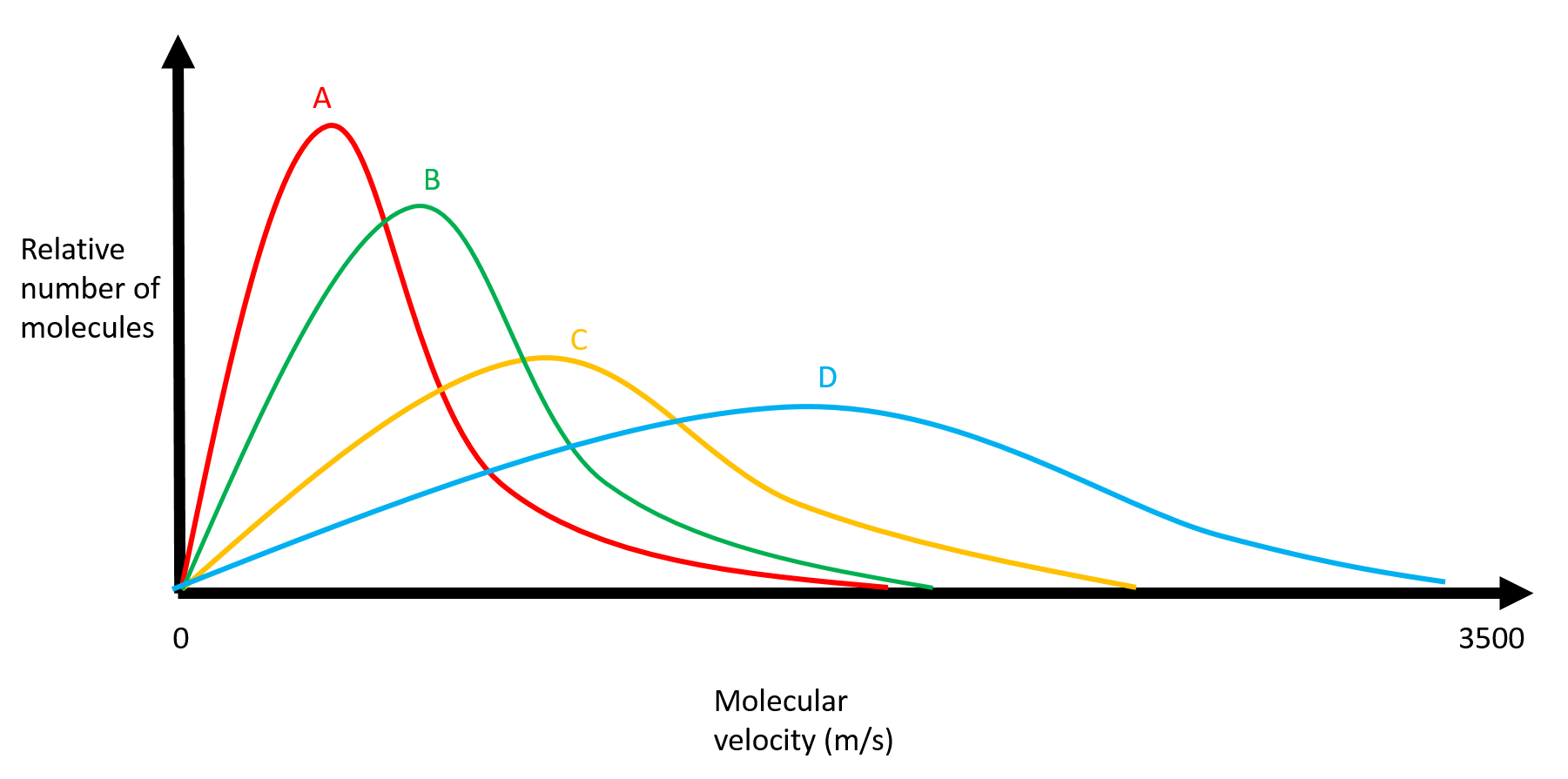B

C

A

D

A

Explanation:

Recall that the kinetic theory of gases states that if molecules are at the same temperature, then the mass of the molecules will determine their velocities. In a mixture of gases at a given temperature, the heavier gases will, on average, travel slower than the lighter ones.

Thus, A must be the gas with the highest molar mass because it has the most molecules traveling at the slowest velocity.

### Example Question #3 : Gases

Which is not characteristic of gases?

Gases expand to fill their containers.

Gases are easy to compress.

The pressure of a gas decreases as more gas is added to the container.

Gases occupy more space than the liquids or solids that they form.

The pressure of a gas decreases as more gas is added to the container.

Explanation:

There is more free space between gas particles than there is between liquid particles, and more free space between liquid particles than there is between solid particles. This makes gases very easy to compress as compared to solids and liquids.

Both liquids and gases can expand to fill their containers because the individual particles can move past each other. This is not possible in a solid because the individual particles are rigidly packed.

Additionally, the volume of a liquid or solid can increase by approximately eight hundred times when it becomes a gas. This large change in volume can be harnessed to do work. For example, a steam engine works when water boils to form a gas (steam), which has a larger volume. Steam can thus escape from the container in which it was produced and perform work.

Lastly, the pressure of a gas increases as more gas is added to the container. Pressure is defined as the force exerted by the gas over a certain area. If more gas particles are added to a fixed area, they will exert a greater force over that area, increasing the overall pressure.

### Example Question #4 : Gases

The Haber process is a common reaction used in the industrial production of ammonia: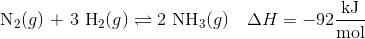Which of the following would increase the production of NH3(g)?

Decreasing volume

Increasing temperature

Increasing volume

Decreasing volume

Explanation:

Let's first look at the affects of adding an inert gas such as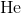. He would not take part in the reaction, so the equation can be written as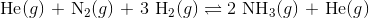Since He is on both sides of the equation, equilibrium is not affected.

Now let's look at the affects of heat. Because the reaction is exothermic, it can be rewritten as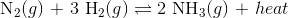Since heat is a product of the reaction, increasing temperature would shift the reaction to the left.

Finally, we know that changing the volume changes the internal pressure of the system. We know that the affect of this change is dependent on the number of moles in the gaseous phase.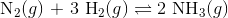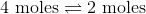In order to increase the production of NH3 (the side with the least number of moles), we need to increase the internal pressure of the system by decreasing the volume.

Decreasing the volume would increase the production of ammonia.